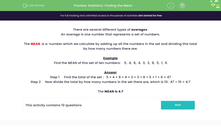# Statistics: Finding the Mean

In this worksheet, students calculate the mean of a given set of data.Key stage:  KS 2

Curriculum topic:   Statistics

Curriculum subtopic:   Calculate Average/Interpret Mean

Popular topics:   Numbers worksheets

Difficulty level:#### Worksheet Overview

There are several different types of averages.

An average is one number that represents a set of numbers.

The MEAN  is a  number which we calculate by adding up all the numbers in the set and dividing this total by how many numbers there are.

Example

Find the MEAN of this set of ten numbers:    5,  4,  8,  4,  3,  3,  8,  5,  1,  6

Step 1     Find the total of the set :   5 + 4 + 8 + 4 + 3 + 3 + 8 + 5 + 1 + 6 = 47

Step 2     Now divide the total by how many numbers in the set there are, which is 10:  47 ÷ 10 = 4.7

The MEAN is 4.7

### What is EdPlace?

We're your National Curriculum aligned online education content provider helping each child succeed in English, maths and science from year 1 to GCSE. With an EdPlace account you’ll be able to track and measure progress, helping each child achieve their best. We build confidence and attainment by personalising each child’s learning at a level that suits them.

Get started